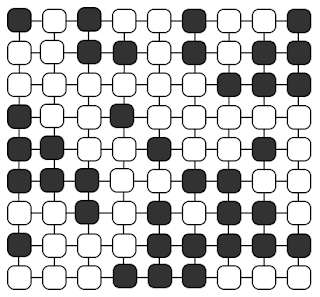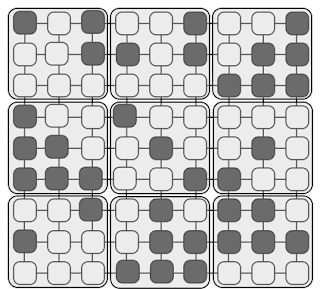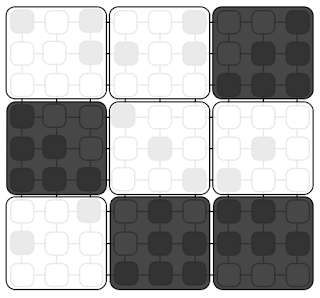A new video which more or less completes the critical phenomena series. Jump straight to it if you want to skip the background.

One of my favourite topics is the critical point. I’ve posted many times on it, so to keep this short you can go back here for a summary. In brief, we’re looking at a small point on the phase diagram where two phases begin to look the same. The correlation length diverges and all hell breaks loose. Well, lots of things diverge. At the critical point all length scales are equivalent and, perhaps most remarkably, microscopic details become almost irrelevant. Different materials fit into a small number of universality classes that share broad properties such as symmetry or dimensionality.

For a long time this universal nature was known about but it couldn’t be said for sure if it was a truly universal thing, or just a really good approximation. Then along came the renormalisation group (RG), which gave a strong theoretical basis to critical phenomena and sorted everything out.

The renormalisation group is usually at the back end of an advanced statistical mechanics course, and that is not the level I’m going for with this blog. However, when making the videos for the demonstration of scale invariance and universality it became apparent that, even just making the pictures for these videos, I had to use RG. Even if I didn’t realise it.

First I’ll try to explain schematically what RG aims to do. Then I’ll show how this is similar to how I make my pictures, and finally we’ll get to a demonstration of RG flow at the end. I’ll try not to dumb it down too much but I also want to be as quick as possible.

#### Renormalisation group#

Let’s look at how we do this with the Ising model. A simple model for a magnet where spins, sigma, (magnetic dipoles) can point up or down, $$\sigma=\pm 1$$, and like to align with their neighbours through a coupling constant, $$J$$. The energy is a sum over nearest neighbour pairs

$$\displaystyle E=\sum_{ij} -J \sigma_i \sigma_j$$

Where RG enters is to say that, if the physics is the same on all length scales, then we should be able to able to rescale our problem, to cast it on a different length scale, and get back the same thing. In real-space RG this is done by blocking. We bunch a group of our spins up together and form a new super spin that takes on the majority value of its constituents. It’s as though the spins in the block get together and vote on how they want to be represented, and then we can deal with them as one.

Here’s what it looks like. Take an Ising model configurationBlock them togetherAnd voteWe’re left with a pixelated version of the first picture. Now here I will slightly deviate from RG as standard. The next step is to ask, if these super spins were a standalone Ising model, what temperature would they have? If our initial system is right on the critical point then the renormalised (blocked) system should have the same temperature because it should look exactly the same – scale invariance. If you’re even slightly off then the apparent temperature, let’s call it $$T_{RG}$$, will flow away from the critical point towards a fixed point.

These fixed points are the ferromagnet (all spins the same, $$T_{RG}=0$$) or the paramagnet (completely random, $$T_{RG} \rightarrow \infty$$) as shown below.Normally RG is done in terms of coupling constants rather than temperature. However, I think in our case temperature is more intuitive.

#### Zooming out#

By now the link between RG and the pictures I make may already be clear. The configurations I will show below are made of something like $$10^{10}$$ spins. Clearly I can’t make a 10 Giga pixel jpeg so I have to compress the data. In fact the way I do it is an almost identical blocking process. Spins are bundled into $$b \times b$$ blocks and I use a contrasting function (a fairly sharp tanh) that is not far away at all from majority rule as described above.

If we start by zooming in to a 768x768 subsection then each pixel is precisely one spin. As we zoom out we eventually need to start blocking spins together. In the video below there are three systems: one ever-so-slightly below $$T_c$$, one ever-so-slightly above $$T_c$$ and one right on the money. At maximum zoom they all look pretty much the same. If you had to guess their temperatures you’d say they’re all critical.

As we start to zoom out we can see structure on length scales, and the apparent temperatures start to change, in fact they flow towards the fixed point phases. Video below, recommend you switch on HD and watch it full screen

So there it is. RG in action. If you’re not precisely on the critical point then you will eventually find a length scale where you clearly have a ferromagnet or a paramagnet. At the critical point itself you can zoom out forever and it will always look the same. The renormalisation group is a really difficult subject, but I hope this visualisation can at least give a feeling for what’s going on, even if the mathematical detail is a bit more challenging.